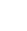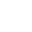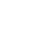HomeModulesArticlesVideosLife EventsCalculatorsQuizJargonExtras

General Assumptions

Assumption A - Month: All months are assumed to be equal. In reality, some loans accrue on a daily basis this can lead to varying interest in different months.

Assumption B - Number of Weeks & Fortnights in a Year: One year is assumed to contain exactly 52 weeks or 26 fortnights. Thus the assumption is for a 364 day year.

Assumption C - Rounding: The calculation uses the unrounded repayment to derive the amount of interest payable over the full term of the loan, however, institutions round repayments to the nearest cent. Unrounded payments provide for constant line within the graph.

Assumption D - Compounded: Interest is compounded on the basis of frequency from the calculator's input fields. In reality, some loan lenders calculate interests on a daily basis this can lead to varying interest in different months.

Assumption E - Timing of Interest Conversion: Assume that interest is charged to the loan account at the same frequency as the repayments are made. In practice, there may be differences between the timing of the loan repayments and the timing of the interest charges being added to the loan balance.

Assumption F - Last Repayment: The final repayment after the increase in repayment amount will be a partial repayment as required to reduce the loan balance to zero without negative owning amount.

Assumption G - Default Living Expense: Number of dependants     Single      Joint

0                     \$13,713   \$16,236

1                     \$16,445   \$19,743

2                     \$19,743   \$22,330

3                     \$22,330   \$25,987

4                     \$25,987   \$28,123

Assumption H - Buffer or Extra Interest: 1.5%. In order to avoid the risk of interest increasing, many lenders use a buffer / extra interest to calculator borrowing power but the repayments and total interests payable are still calculated without buffer rate.

Calculators Loan Repayment Calculator: Calculate what the minimum weekly, fortnightly or monthly loan repayments would be for any borrowed amount Assumption A, B, C, D, E.

Extra Repayment Calculator: Find out how much a borrower can save in interest or shorten the length of your loan term by making additional repayments over and above your current regular repayments. Assumption A, B, C, D, E, F.

Lump Sum Repayment Calculator: Find out how much a borrower can save in interest or shorten the length of your loan term by making an additional repayment over and above your current regular repayments. Assumption A, B, C, D, E, F.

Split Loan Calculator: Splitting a home loan into fixed and variable rate portions can provide insurance against future changes in interest rates that no one can predict. This calculator calculates your repayments and total interest under different fixed and variable rate scenarios. Assumption A, B, C, D, E, F.

How Long to Repay Calculator: Find out how long it would take to repay a loan by paying different amounts. Assumption A, B, C, D, E, F.

Honeymoon Loan Calculator: Calculate what Home Loan Repayments would be with an Introductory or Honeymoon Interest Rate. Assumption A, B, C, D, E.

Home Loan Offset Calculator: Find out how much interest and loan term a borrower can save by utilising a Home Loan Offset Account. Assumption A, B, C, D, E, F.

Comparison Rate Calculator: Calculate the comparison rate of a loan. Assumption A, B, C, D, E.

Loan Comparison Calculator: Select any two home loans on the market for a direct cost comparison. Determine which is the cheapest in total fees and interest over the life of the loan. Assumption A, B, C, D, E.

Borrowing Power Calculator: Find out how much you can expect to borrow for a home based on your current salary and existing financial commitments. Assumption A, B, C, D, E, G, H. Other Assumptions see the Assumption tab on the Borrowing Power calculator.

Reverse Mortgage Calculator: Calculate how much you could borrow with a Reverse Mortgage Loan and find out when the value of a home will become zero or protected level. Assumption A, C, D, E. Other Assumptions see the Assumption tab on the Reverse Mortgage calculator.

Credit Card Calculator: Calculate how long it will take a borrower to repay his credit card debt and how extra repayments will help. Assumption A, C, D, E.

Stamp Duty Calculator: Calculate how much stamp duty will be payable on the purchase of a new property. The rates and formulae can be found on each state's revenue Office. See the links on the calculator. Property Buying / Selling Cost Calculators: Calculate how much it will cost a property buyer or seller to buy or sell a property including Government Charges. The government rates and formulae can be found on each state's revenue Office. See the links on the stamp duty calculator.

Budget Planner: Enter the annual income and expenses to help assess a person's financial position. Formula: Surplus = All Income – All Expense.

Income Tax Calculator: Calculate a simple income tax liability according to the ATO's personal income tax scales. See ATO web site for tax rate.

Leasing Calculator: Calculate how much your lease will cost you over the length of the term. Assumption A, C, D, E.

Saving Calculator: Calculate the total accumulation in a savings account with a regular deposit arrangement. Assumption A, C, D, E.

Term Deposit Calculator: Calculate the total accumulated balance and interest components in a term deposit account. Assumption A, C. Assumption I: Interests are compounded yearly, monthly and daily respectively. If compounded daily, assume 'annual interest / 365' as daily interest and assume there are 30 days in each month.